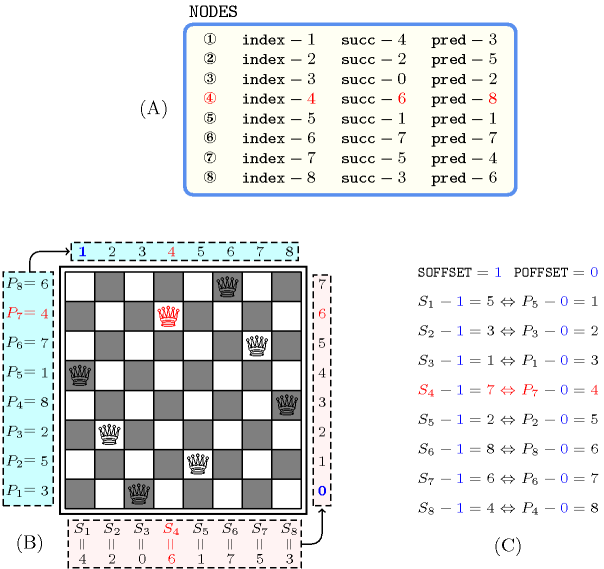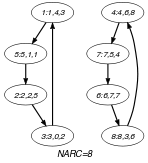## 5.200. inverse_offset

Origin

Gecode

Constraint

$\mathrm{𝚒𝚗𝚟𝚎𝚛𝚜𝚎}_\mathrm{𝚘𝚏𝚏𝚜𝚎𝚝}\left(\mathrm{𝚂𝙾𝙵𝙵𝚂𝙴𝚃},\mathrm{𝙿𝙾𝙵𝙵𝚂𝙴𝚃},\mathrm{𝙽𝙾𝙳𝙴𝚂}\right)$

Synonym

$\mathrm{𝚌𝚑𝚊𝚗𝚗𝚎𝚕}$.

Arguments
 $\mathrm{𝚂𝙾𝙵𝙵𝚂𝙴𝚃}$ $\mathrm{𝚒𝚗𝚝}$ $\mathrm{𝙿𝙾𝙵𝙵𝚂𝙴𝚃}$ $\mathrm{𝚒𝚗𝚝}$ $\mathrm{𝙽𝙾𝙳𝙴𝚂}$ $\mathrm{𝚌𝚘𝚕𝚕𝚎𝚌𝚝𝚒𝚘𝚗}\left(\mathrm{𝚒𝚗𝚍𝚎𝚡}-\mathrm{𝚒𝚗𝚝},\mathrm{𝚜𝚞𝚌𝚌}-\mathrm{𝚍𝚟𝚊𝚛},\mathrm{𝚙𝚛𝚎𝚍}-\mathrm{𝚍𝚟𝚊𝚛}\right)$
Restrictions
 $\mathrm{𝚛𝚎𝚚𝚞𝚒𝚛𝚎𝚍}$$\left(\mathrm{𝙽𝙾𝙳𝙴𝚂},\left[\mathrm{𝚒𝚗𝚍𝚎𝚡},\mathrm{𝚜𝚞𝚌𝚌},\mathrm{𝚙𝚛𝚎𝚍}\right]\right)$ $\mathrm{𝙽𝙾𝙳𝙴𝚂}.\mathrm{𝚒𝚗𝚍𝚎𝚡}\ge 1$ $\mathrm{𝙽𝙾𝙳𝙴𝚂}.\mathrm{𝚒𝚗𝚍𝚎𝚡}\le |\mathrm{𝙽𝙾𝙳𝙴𝚂}|$ $\mathrm{𝚍𝚒𝚜𝚝𝚒𝚗𝚌𝚝}$$\left(\mathrm{𝙽𝙾𝙳𝙴𝚂},\mathrm{𝚒𝚗𝚍𝚎𝚡}\right)$ $\mathrm{𝙽𝙾𝙳𝙴𝚂}.\mathrm{𝚜𝚞𝚌𝚌}\ge 1+\mathrm{𝚂𝙾𝙵𝙵𝚂𝙴𝚃}$ $\mathrm{𝙽𝙾𝙳𝙴𝚂}.\mathrm{𝚜𝚞𝚌𝚌}\le |\mathrm{𝙽𝙾𝙳𝙴𝚂}|+\mathrm{𝚂𝙾𝙵𝙵𝚂𝙴𝚃}$ $\mathrm{𝙽𝙾𝙳𝙴𝚂}.\mathrm{𝚙𝚛𝚎𝚍}\ge 1+\mathrm{𝙿𝙾𝙵𝙵𝚂𝙴𝚃}$ $\mathrm{𝙽𝙾𝙳𝙴𝚂}.\mathrm{𝚙𝚛𝚎𝚍}\le |\mathrm{𝙽𝙾𝙳𝙴𝚂}|+\mathrm{𝙿𝙾𝙵𝙵𝚂𝙴𝚃}$
Purpose

Enforce each vertex of a digraph to have exactly one predecessor and one successor. In addition the following two statements are equivalent:

1. The successor of the ${i}^{th}$ node minus $\mathrm{𝐒𝐎𝐅𝐅𝐒𝐄𝐓}$ is equal to $j$.

2. The predecessor of the ${j}^{th}$ node minus $\mathrm{𝐏𝐎𝐅𝐅𝐒𝐄𝐓}$ is equal to $i$.

I.e., $\mathrm{𝙽𝙾𝙳𝙴𝚂}\left[i\right].\mathrm{𝚜𝚞𝚌𝚌}-\mathrm{𝐒𝐎𝐅𝐅𝐒𝐄𝐓}=j⇔\mathrm{𝙽𝙾𝙳𝙴𝚂}\left[j\right].\mathrm{𝚙𝚛𝚎𝚍}-\mathrm{𝐏𝐎𝐅𝐅𝐒𝐄𝐓}=i$.

Example
$\left(\begin{array}{c}-1,0,〈\begin{array}{ccc}\mathrm{𝚒𝚗𝚍𝚎𝚡}-1\hfill & \mathrm{𝚜𝚞𝚌𝚌}-4\hfill & \mathrm{𝚙𝚛𝚎𝚍}-3,\hfill \\ \mathrm{𝚒𝚗𝚍𝚎𝚡}-2\hfill & \mathrm{𝚜𝚞𝚌𝚌}-2\hfill & \mathrm{𝚙𝚛𝚎𝚍}-5,\hfill \\ \mathrm{𝚒𝚗𝚍𝚎𝚡}-3\hfill & \mathrm{𝚜𝚞𝚌𝚌}-0\hfill & \mathrm{𝚙𝚛𝚎𝚍}-2,\hfill \\ \mathrm{𝚒𝚗𝚍𝚎𝚡}-4\hfill & \mathrm{𝚜𝚞𝚌𝚌}-6\hfill & \mathrm{𝚙𝚛𝚎𝚍}-8,\hfill \\ \mathrm{𝚒𝚗𝚍𝚎𝚡}-5\hfill & \mathrm{𝚜𝚞𝚌𝚌}-1\hfill & \mathrm{𝚙𝚛𝚎𝚍}-1,\hfill \\ \mathrm{𝚒𝚗𝚍𝚎𝚡}-6\hfill & \mathrm{𝚜𝚞𝚌𝚌}-7\hfill & \mathrm{𝚙𝚛𝚎𝚍}-7,\hfill \\ \mathrm{𝚒𝚗𝚍𝚎𝚡}-7\hfill & \mathrm{𝚜𝚞𝚌𝚌}-5\hfill & \mathrm{𝚙𝚛𝚎𝚍}-4,\hfill \\ \mathrm{𝚒𝚗𝚍𝚎𝚡}-8\hfill & \mathrm{𝚜𝚞𝚌𝚌}-3\hfill & \mathrm{𝚙𝚛𝚎𝚍}-6\hfill \end{array}〉\hfill \end{array}\right)$

The $\mathrm{𝚒𝚗𝚟𝚎𝚛𝚜𝚎}_\mathrm{𝚘𝚏𝚏𝚜𝚎𝚝}$ constraint holds since:

• $\mathrm{𝙽𝙾𝙳𝙴𝚂}\left[1\right].\mathrm{𝚜𝚞𝚌𝚌}-\left(-1\right)=5⇔\mathrm{𝙽𝙾𝙳𝙴𝚂}\left[5\right].\mathrm{𝚙𝚛𝚎𝚍}-0=1$,

• $\mathrm{𝙽𝙾𝙳𝙴𝚂}\left[2\right].\mathrm{𝚜𝚞𝚌𝚌}-\left(-1\right)=3⇔\mathrm{𝙽𝙾𝙳𝙴𝚂}\left[3\right].\mathrm{𝚙𝚛𝚎𝚍}-0=2$,

• $\mathrm{𝙽𝙾𝙳𝙴𝚂}\left[3\right].\mathrm{𝚜𝚞𝚌𝚌}-\left(-1\right)=1⇔\mathrm{𝙽𝙾𝙳𝙴𝚂}\left[1\right].\mathrm{𝚙𝚛𝚎𝚍}-0=3$,

• $\mathrm{𝙽𝙾𝙳𝙴𝚂}\left[4\right].\mathrm{𝚜𝚞𝚌𝚌}-\left(-1\right)=7⇔\mathrm{𝙽𝙾𝙳𝙴𝚂}\left[7\right].\mathrm{𝚙𝚛𝚎𝚍}-0=4$,

• $\mathrm{𝙽𝙾𝙳𝙴𝚂}\left[5\right].\mathrm{𝚜𝚞𝚌𝚌}-\left(-1\right)=2⇔\mathrm{𝙽𝙾𝙳𝙴𝚂}\left[2\right].\mathrm{𝚙𝚛𝚎𝚍}-0=5$.

• $\mathrm{𝙽𝙾𝙳𝙴𝚂}\left[6\right].\mathrm{𝚜𝚞𝚌𝚌}-\left(-1\right)=8⇔\mathrm{𝙽𝙾𝙳𝙴𝚂}\left[8\right].\mathrm{𝚙𝚛𝚎𝚍}-0=6$.

• $\mathrm{𝙽𝙾𝙳𝙴𝚂}\left[7\right].\mathrm{𝚜𝚞𝚌𝚌}-\left(-1\right)=6⇔\mathrm{𝙽𝙾𝙳𝙴𝚂}\left[6\right].\mathrm{𝚙𝚛𝚎𝚍}-0=7$.

• $\mathrm{𝙽𝙾𝙳𝙴𝚂}\left[8\right].\mathrm{𝚜𝚞𝚌𝚌}-\left(-1\right)=4⇔\mathrm{𝙽𝙾𝙳𝙴𝚂}\left[4\right].\mathrm{𝚙𝚛𝚎𝚍}-0=8$.

Figure 5.200.1 shows the board that can be associated with this example.

##### Figure 5.200.1. Example slot where we highlight the fourth item in red showing the relation between ${S}_{4}$ and ${P}_{7}$, where ${S}_{i}$ and ${P}_{i}$ (with $1\le i\le 8$) respectively stands for the successor and predecessor attributes of the ${i}^{\mathrm{𝚝𝚑}}$ item of the $\mathrm{𝙽𝙾𝙳𝙴𝚂}$ collection (A) Collection of nodes passed to the $\mathrm{𝚒𝚗𝚟𝚎𝚛𝚜𝚎}_\mathrm{𝚘𝚏𝚏𝚜𝚎𝚝}$ constraint, (B) Corresponding board, (C) Conditions linking the successor and the predecessor attributes via the offsets $\mathrm{𝚂𝙾𝙵𝙵𝚂𝙴𝚃}=1$ and $\mathrm{𝙿𝙾𝙵𝙵𝚂𝙴𝚃}=0$.Typical
 $\mathrm{𝚂𝙾𝙵𝙵𝚂𝙴𝚃}\ge -1$ $\mathrm{𝚂𝙾𝙵𝙵𝚂𝙴𝚃}\le 1$ $\mathrm{𝙿𝙾𝙵𝙵𝚂𝙴𝚃}\ge -1$ $\mathrm{𝙿𝙾𝙵𝙵𝚂𝙴𝚃}\le 1$ $|\mathrm{𝙽𝙾𝙳𝙴𝚂}|>1$
Symmetry

Items of $\mathrm{𝙽𝙾𝙳𝙴𝚂}$ are permutable.

Arg. properties
• Functional dependency: $\mathrm{𝙽𝙾𝙳𝙴𝚂}.\mathrm{𝚜𝚞𝚌𝚌}$ determined by $\mathrm{𝚂𝙾𝙵𝙵𝚂𝙴𝚃}$, $\mathrm{𝙿𝙾𝙵𝙵𝚂𝙴𝚃}$, $\mathrm{𝙽𝙾𝙳𝙴𝚂}.\mathrm{𝚒𝚗𝚍𝚎𝚡}$ and $\mathrm{𝙽𝙾𝙳𝙴𝚂}.\mathrm{𝚙𝚛𝚎𝚍}$.

• Functional dependency: $\mathrm{𝙽𝙾𝙳𝙴𝚂}.\mathrm{𝚙𝚛𝚎𝚍}$ determined by $\mathrm{𝚂𝙾𝙵𝙵𝚂𝙴𝚃}$, $\mathrm{𝙿𝙾𝙵𝙵𝚂𝙴𝚃}$, $\mathrm{𝙽𝙾𝙳𝙴𝚂}.\mathrm{𝚒𝚗𝚍𝚎𝚡}$ and $\mathrm{𝙽𝙾𝙳𝙴𝚂}.\mathrm{𝚜𝚞𝚌𝚌}$.

Remark

The $\mathrm{𝚒𝚗𝚟𝚎𝚛𝚜𝚎}_\mathrm{𝚘𝚏𝚏𝚜𝚎𝚝}$ constraint is called $\mathrm{𝚌𝚑𝚊𝚗𝚗𝚎𝚕}$ in Gecode (http://www.gecode.org/). Having two offsets was motivated by the fact that it is possible to declare arrays at any position in the MiniZinc modelling language.

Systems

specialisation: $\mathrm{𝚒𝚗𝚟𝚎𝚛𝚜𝚎}$ (assume that $\mathrm{𝚂𝙾𝙵𝙵𝚂𝙴𝚃}$ and $\mathrm{𝙿𝙾𝙵𝙵𝚂𝙴𝚃}$ are both equal to 0).

Keywords
Arc input(s)

$\mathrm{𝙽𝙾𝙳𝙴𝚂}$

Arc generator
$\mathrm{𝐶𝐿𝐼𝑄𝑈𝐸}$$↦\mathrm{𝚌𝚘𝚕𝚕𝚎𝚌𝚝𝚒𝚘𝚗}\left(\mathrm{𝚗𝚘𝚍𝚎𝚜}\mathtt{1},\mathrm{𝚗𝚘𝚍𝚎𝚜}\mathtt{2}\right)$

Arc arity
Arc constraint(s)
 $•\mathrm{𝚗𝚘𝚍𝚎𝚜}\mathtt{1}.\mathrm{𝚜𝚞𝚌𝚌}-\mathrm{𝚂𝙾𝙵𝙵𝚂𝙴𝚃}=\mathrm{𝚗𝚘𝚍𝚎𝚜}\mathtt{2}.\mathrm{𝚒𝚗𝚍𝚎𝚡}$ $•\mathrm{𝚗𝚘𝚍𝚎𝚜}\mathtt{2}.\mathrm{𝚙𝚛𝚎𝚍}-\mathrm{𝙿𝙾𝙵𝙵𝚂𝙴𝚃}=\mathrm{𝚗𝚘𝚍𝚎𝚜}\mathtt{1}.\mathrm{𝚒𝚗𝚍𝚎𝚡}$
Graph property(ies)
$\mathrm{𝐍𝐀𝐑𝐂}$$=|\mathrm{𝙽𝙾𝙳𝙴𝚂}|$

Graph model

In order to express the binary constraint that links two vertices one has to make explicit the identifier of the vertices. This is why the $\mathrm{𝚒𝚗𝚟𝚎𝚛𝚜𝚎}_\mathrm{𝚘𝚏𝚏𝚜𝚎𝚝}$ constraint considers objects that have three attributes:

• One fixed attribute $\mathrm{𝚒𝚗𝚍𝚎𝚡}$ that is the identifier of the vertex,

• One variable attribute $\mathrm{𝚜𝚞𝚌𝚌}$ that is the successor of the vertex,

• One variable attribute $\mathrm{𝚙𝚛𝚎𝚍}$ that is the predecessor of the vertex.

Parts (A) and (B) of Figure 5.200.2 respectively show the initial and final graph associated with the Example slot. Since we use the $\mathrm{𝐍𝐀𝐑𝐂}$ graph property, the arcs of the final graph are stressed in bold.

##### Figure 5.200.2. Initial and final graph of the $\mathrm{𝚒𝚗𝚟𝚎𝚛𝚜𝚎}_\mathrm{𝚘𝚏𝚏𝚜𝚎𝚝}$ constraint(a) (b)